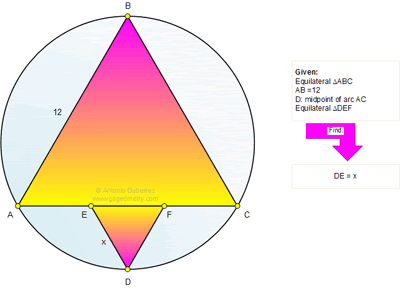## Monday, December 5, 2011

### Problem 699: Equilateral Triangle, Circle, Circular Segment, Midpoint, Metric Relations

Geometry Problem
Level: Mathematics Education, High School, Honors Geometry, College.

Click the figure below to see the complete problem 699.1.http://img821.imageshack.us/img821/6871/problem699.png
BD cut EF at G ( see picture)
Note that DG=OG (angle ABC=60)
Triangle ABG similar to tri. EDG with ratio =BG/DG=3
So x= AB/3= 4
Peter Tran

2.DAC = 30, AED = 120 imply ADE = 30
So AE = ED = x
FC = DF = x (by symmetry)
So x = AE = EF = FC = AC/3 = 4